M358K 2nd Midterm Exam Solutions.  October 31, 2001

Note: these solutions were computed without a calculator, so they may differ in the 3rd or 4th decimal from more precise answers.

1. True or false. (2 pages) If the statement is false, explain why (e.g., give an example where the statement fails).

a) The p-value is the probability, assuming the null hypothesis is false, that the test statistic will take a value at least as extreme as that actually observed.

False. See (b) for the correct definition.

b) The p-value is the probability, assuming the null hypothesis is true, that the test statistic will take a value at least as extreme as that actually observed.

True.

c) The p-value is the probability that the null hypothesis is true.

False. Other evidence may apply. See (b) for the correct definition.

For questions d-f, suppose that a 99% confidence interval for the mean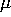of a distribution goes from 17.24 to 23.1.

d) There is a 99% probability that the mean lies between 17.24 and 23.1.

False. There is a 99% probability of a sample mean lying within 2.93 of the true mean, but we don't know what the true mean IS. See (f).

e) If you collect a SRS and compute the mean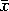, you have a 99% chance of landing between 17.24 and 23.1.

False. This probability depends on whatactually is. If we got lucky with our confidence interval, thenwill have close to a 99% chance on landing there, but if we happened to get a bad interval, then the chances are much less.

f) If you collect a large number of SRSs and compute 99% confidence intervals from each sample, then the true meanwill be found in approximately 99% of these intervals.

True.

g) If the null hypothesis is rejected with 95% confidence, then there is a 5% chance that the null hypothesis is true.

False. Either the null hypothesis is false, or there was a 1-in-20 coincidence. Without additional information you cannot say which is more likely.

The remaining questions refer to a (hypothetical!) court that relies entirely on statistical evidence to determine whether a defendant is innocent or guilty. The null hypothesis is that the defendant is innocent, the court demands a 95% level of significance to convict, and the test has a power of 75%.

h) If the defendant is innocent, he has a 95% chance of being acquitted.

True.

i) If he is guilty, he has a 95% chance of being convicted.

False. Confidence refers to the likelihood of type I errors, not type II errors.

j) If he is guilty, he has a 75% chance of being convicted.

True.

2. Confidence intervals:

Pecans from a given tree have a mean weightwith a standard deviation of 3.5 grams. You collect a SRS of 50 pecans, and measure their mean weightto be 10.4 grams.

a) Construct a 90% confidence interval for the mean.

The distribution offor a givenhas a mean ofand a standard deviation of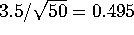grams. We are 90% confident thatlies within 1.645 standard deviations of, so we say, with 90% confidence, thatlies within 1.645 standard deviations of. (There is a 5% chance of being more than 1.645 standard deviations too high and a 5% chance of being that far too low).

Interval is 10.4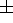0.814 grams, or from 9.586 to 11.214 grams.

b) Construct a 99% confidence interval.

Instead of 1.645 standard deviations, we want 2.575 standard deviations, since F(2.575)=0.995. (We do NOT want F(z)=0.99 - that will give us 98% confidence). 2.575 standard deviations is 1.225 grams, so

Interval is 10.41.225 grams, or from 9.175 to 11.625 grams.

3. Testing Light bulbs

A manufacturer claims that 70% of its light bulbs last 300 hours or more. You take a SRS of 100 light bulbs and test them. Of you sample, 63 last 300 hours or more, while 37 do not.

a) State the null hypothesis and the alternative hypothesis.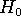is that 70% of the light bulbs last 300 hours or more, i.e. that the probability of a randomly chosen light bulb lasting 300 hours or more is 0.7.

A 2-sided alternative is that the probability is NOT 0.7.

A 1-sided alternative is that the probability is less than 0.7.

If you're accusing the manufacturer of overstating the quality of his light bulbs, then the 1-sided alternative makes more sense. If you're accusing him of inaccuracy, then you want the 2-sided alternative. For exam purposes, I'll accept either one, as long as you stated it unambiguously.

c) What is the p-value?

Checking 100 light bulbs is a binomial process, with n=100 and p=0.7. The mean (assumingtrue) is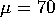and the standard deviation is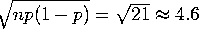. We use the normal approximation. The probability of getting 63 or fewer is (by the continuity correction) the area under the curve to the left of 63.5, whose z-score is (63.5 - 70)/4.6 = -1.41. This area is 0.0808.

If we are using a 1-sided alternative, the p-value is 0.0808, or about 8%.

For a 2-sided alternative the p-value is double that, or 16.16%.

b) Can you conclude, with 90% confidence, that the manufacturer's claims are false?

For the 1-sided alternative hypothesis, yes, since 0.0808 < 0.1. However, if you are using a 2-sided alternative hypothesis, the answer is no, since 0.1616 > 0.1.

4. Random drug tests:

A laboratory is testing drugs to see if they have harmful side effects. For each drug, the laboratory runs a clinical test on a population of mice, notes how many get sick, and does statistical calculations. In each case, the null hypothesis of ``drug is harmless'' if the mice show symptoms at an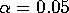level of significance.

Suppose that 1000 drugs are tested, of which 900 truly are safe, while 100 are dangerous. (You can assume that what's OK for mice is OK for people, and vice-versa)

a) About how many safe drugs is the laboratory likely to reject?

Since we are working at an 0.05 level of significance, the likelihood of a safe drug being rejected is 5%. 5% of 900 is 45, so we expect about 45 safe drugs to be rejected.

b) Suppose that the power of each test is 85%. About how many dangerous drugs is the laboratory likely to approve?

The power is the likelihood of rejecting a dangerous drug. 85% of the dangerous drugs are rejected, but 15% are not. 15% of 100 is 15, so about 15 dangerous drugs will be approved.

c) Of the rejected drugs, what fraction are actually safe?

On average, there are 45 rejected safe drugs and 85 rejected dangerous drugs, so the answer is 45/(45+85)=45/130=9/26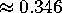.